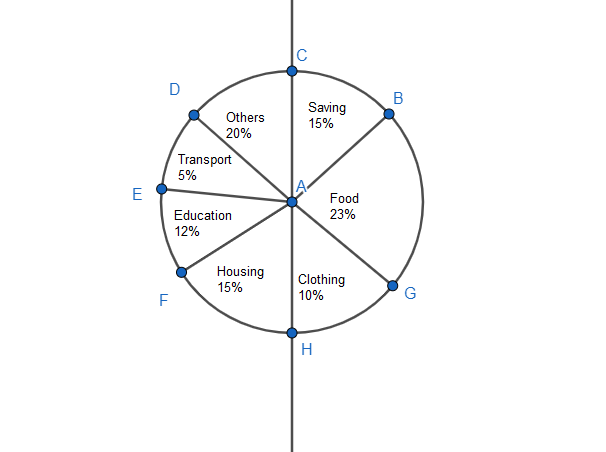Courses
Courses for Kids
Free study material
Offline Centres
MoreLast updated date: 05th Dec 2023
Total views: 280.8k
Views today: 2.80k

# Find the ratio of total amount of money spent on housing to that spent on education wasA. 5:2B. 2:5C. 4:5D. 5:4Verified
280.8k+ views
Hint: We assume the total amount of money spent and also the individual amount of expenditure for each sector. We then find the ratio of total amount of money spent on housing to that spent on education.

Complete step-by-step solution:
Let us assume the total expenditure to be $100x$.
The respective percentages for food, clothing, saving, transportation, education, housing and others are 23, 10, 15, 5, 12, 15 and 20 respectively.
We know for any arbitrary percentage value of a%, we can write it as $\dfrac{a}{100}$. The percentage is to find the respective value out of 100.
Therefore, the amount spent on education is $100x\times \dfrac{12}{100}=12x$ and the amount spent on education is $100x\times \dfrac{15}{100}=15x$.
We now find the ratio of total amount of money spent on housing to that spent on education which is the ratio of $15x$ to $12x$.
The ratio becomes $\dfrac{15x}{12x}=5:4$
The correct option is D.

Note: We can also use the total percentage as the base of the calculation. The total percentage gives $23+10+15+5+12+15+20=100$. That covers the whole circle which helps in getting the fractional value and ratio.